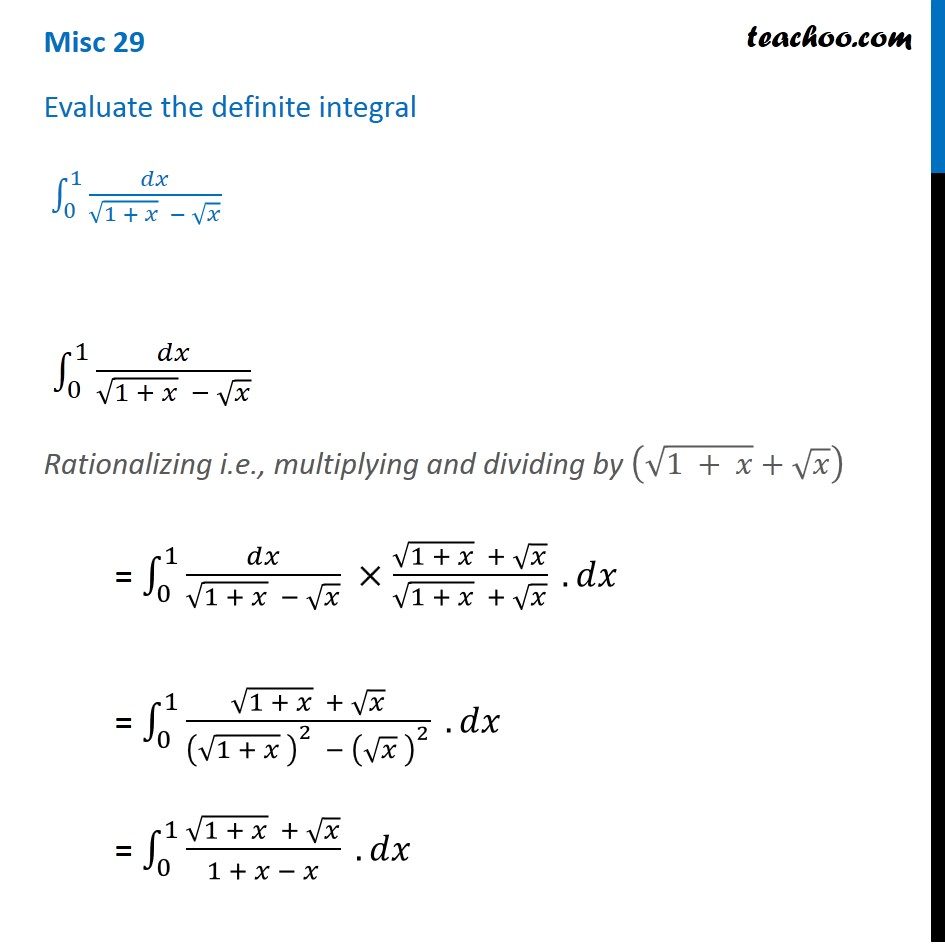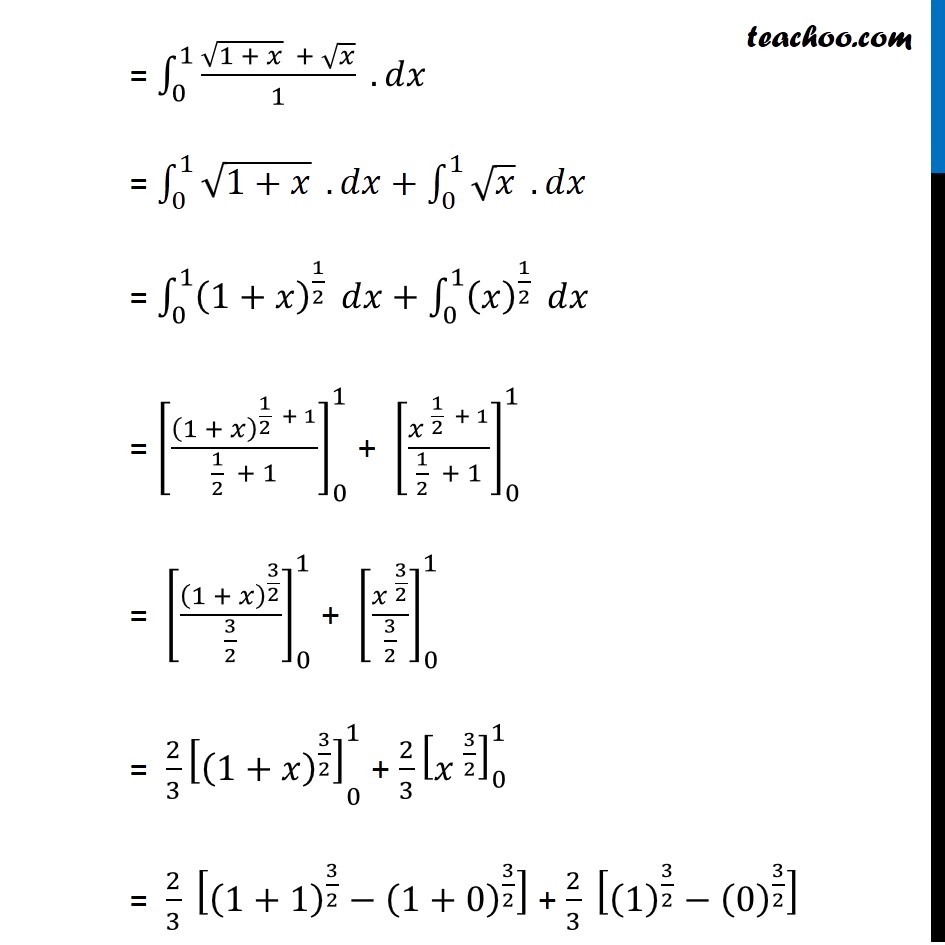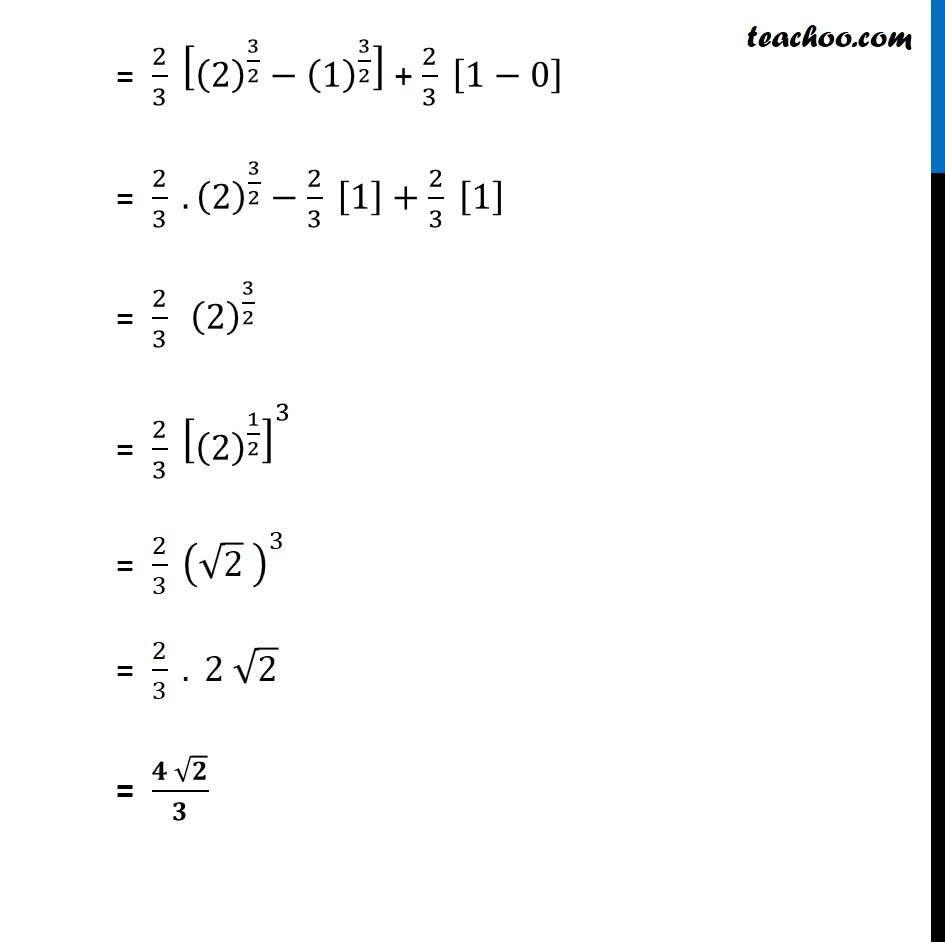Integration Full Chapter Explained - Integration Class 12 - Everything you need1. Chapter 7 Class 12 Integrals
2. Concept wise
3. Definite Integration - By Formulae

Transcript

Misc 29 Evaluate the definite integral ∫_0^1▒〖𝑑𝑥/(√(1 + 𝑥) − √𝑥) 〗 ∫_0^1▒〖𝑑𝑥/(√(1 + 𝑥) − √𝑥) 〗 Rationalizing i.e., multiplying and dividing by (√(1 + 𝑥)+√𝑥) = ∫_0^1▒〖𝑑𝑥/(√(1 + 𝑥) − √𝑥) 〗×(√(1 + 𝑥) + √𝑥)/(√(1 + 𝑥) + √𝑥) . 𝑑𝑥 = ∫_0^1▒(√(1 + 𝑥) + √𝑥)/((√(1 + 𝑥) )^2 − (√𝑥 )^2 ) . 𝑑𝑥 = ∫_0^1▒(√(1 + 𝑥) + √𝑥)/(1 + 𝑥 − 𝑥) . 𝑑𝑥 = ∫_0^1▒(√(1 + 𝑥) + √𝑥)/1 . 𝑑𝑥 = ∫_0^1▒√(1+𝑥) . 𝑑𝑥+∫_0^1▒√𝑥 . 𝑑𝑥" " = ∫_0^1▒(1+𝑥)^(1/2) 𝑑𝑥+∫_0^1▒(𝑥)^(1/2) 𝑑𝑥" " = [(1 + 𝑥)^(1/2 + 1)/(1/2 + 1)]_0^1 + [〖𝑥 〗^(1/2 + 1)/(1/2 + 1)]_0^1 = [(1 + 𝑥)^(3/2)/(3/2)]_0^1 + [〖𝑥 〗^(3/2)/(3/2)]_0^1 = 〖2/3 [(1+𝑥)^(3/2) ]〗_0^1 + 2/3 [〖𝑥 〗^(3/2) ]_0^1 = 2/3 [(1+1)^(3/2)−(1+0)^(3/2) ] + 2/3 [(1)^(3/2)−(0)^(3/2) ] = 2/3 [(2)^(3/2)−(1)^(3/2) ] + 2/3 [1−0] = 2/3 . (2)^(3/2)−2/3 +2/3  = 2/3 (2)^(3/2) = 2/3 [(2)^(1/2) ]^3 = 2/3 (√2 )^3 = 2/3 . 2 √2 = (𝟒 √𝟐)/𝟑

Definite Integration - By Formulae

About the AuthorDavneet Singh
Davneet Singh is a graduate from Indian Institute of Technology, Kanpur. He has been teaching from the past 9 years. He provides courses for Maths and Science at Teachoo.